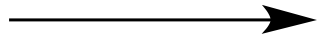Illustrated Glossary of Organic Chemistry

ΔH: The difference in enthalpy between two systems. Can be roughly estimated as the difference in bond energies between the two systems.

 CH4 + 2 O2CO2 + 2 H2O
By definition, ΔH = H(products) - H(reactants). So for the combustion of methane, ΔH = [H(CO2) + 2 H(H2O)] - [H(CH4) - 2 H(O2)].